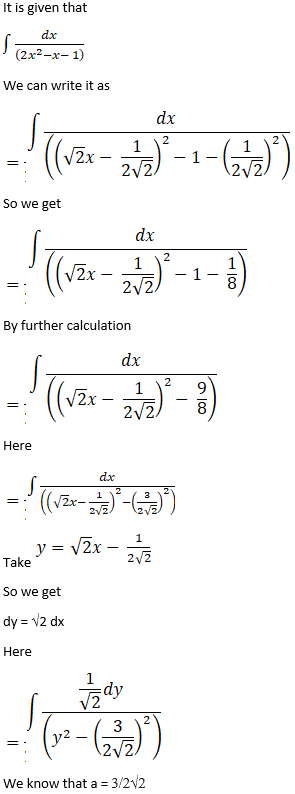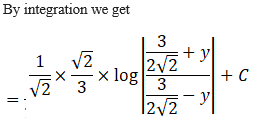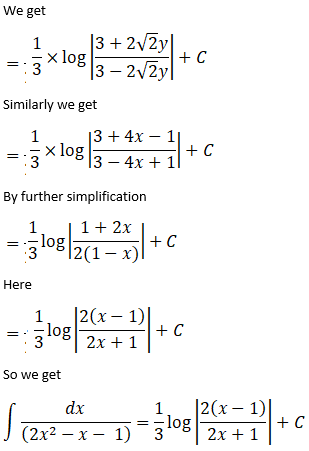# RS Aggarwal Solutions for Class 12 Maths Chapter 14: Some Special Integrals Exercise 14A

Three Special Integrals are the topics which are discussed under the Exercise 14A of Chapter 14. The solutions are crafted in simple language, which matches the understanding abilities of students. Both chapter wise and exercise wise problems can be downloaded effortlessly by the students to boost their exam preparation. All the problems are curated by our experts at BYJU’S for the benefit of Class 12 students. In order to help students score well in the board exam, RS Aggarwal Solutions Class 12 Maths Chapter 14 Some Special Integrals Exercise 14A, PDF links are given here for free download.

## RS Aggarwal Solutions for Class 12 Maths Chapter 14: Some Special Integrals Exercise 14A Download PDF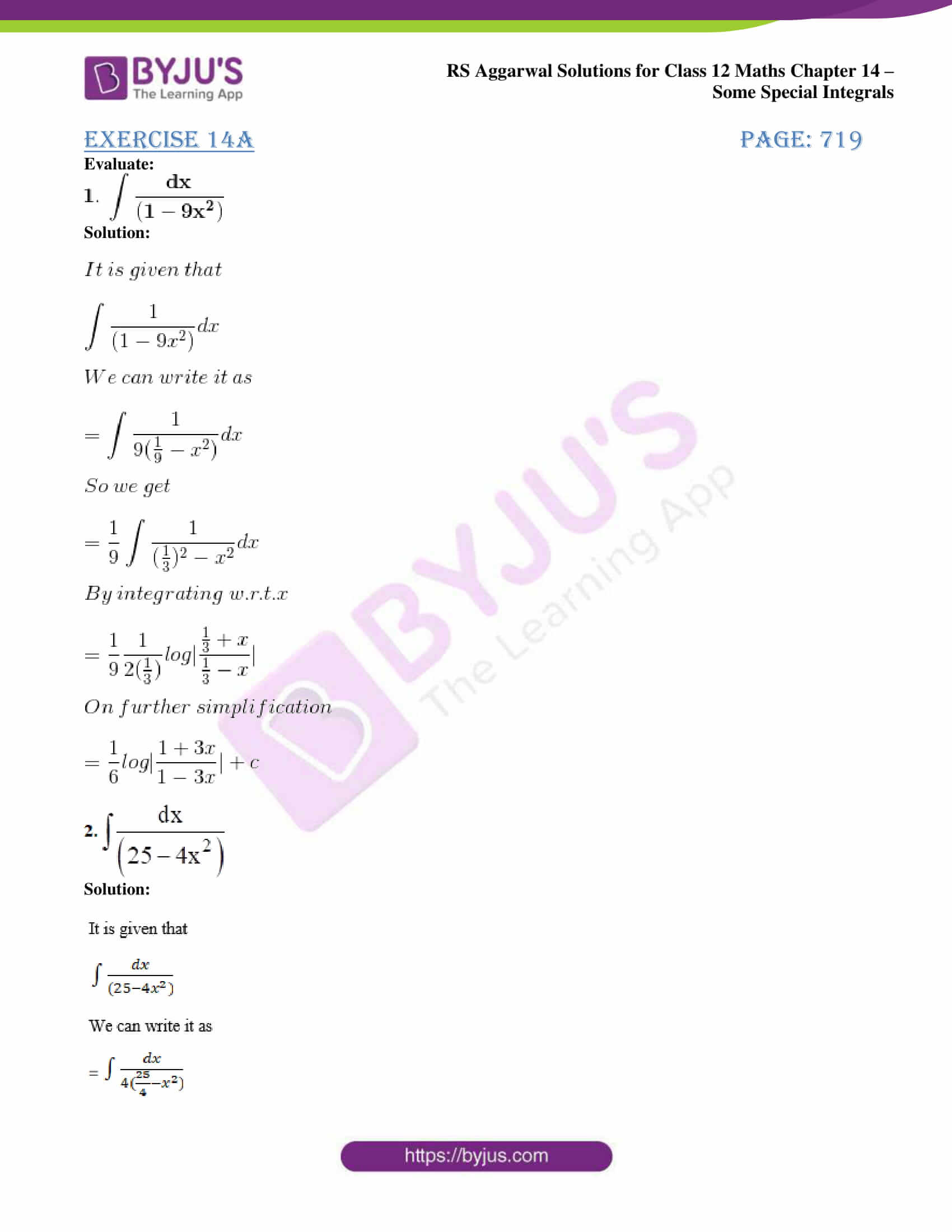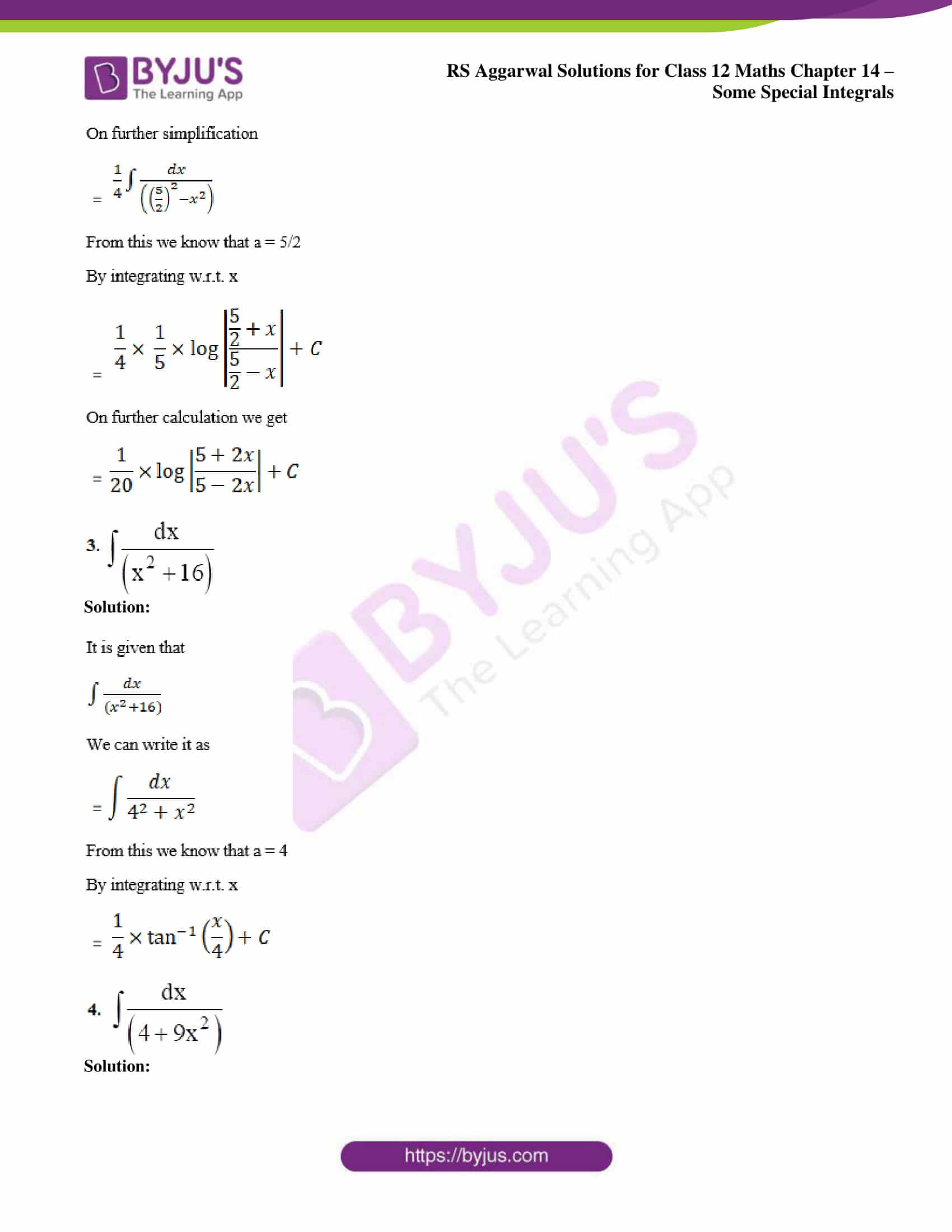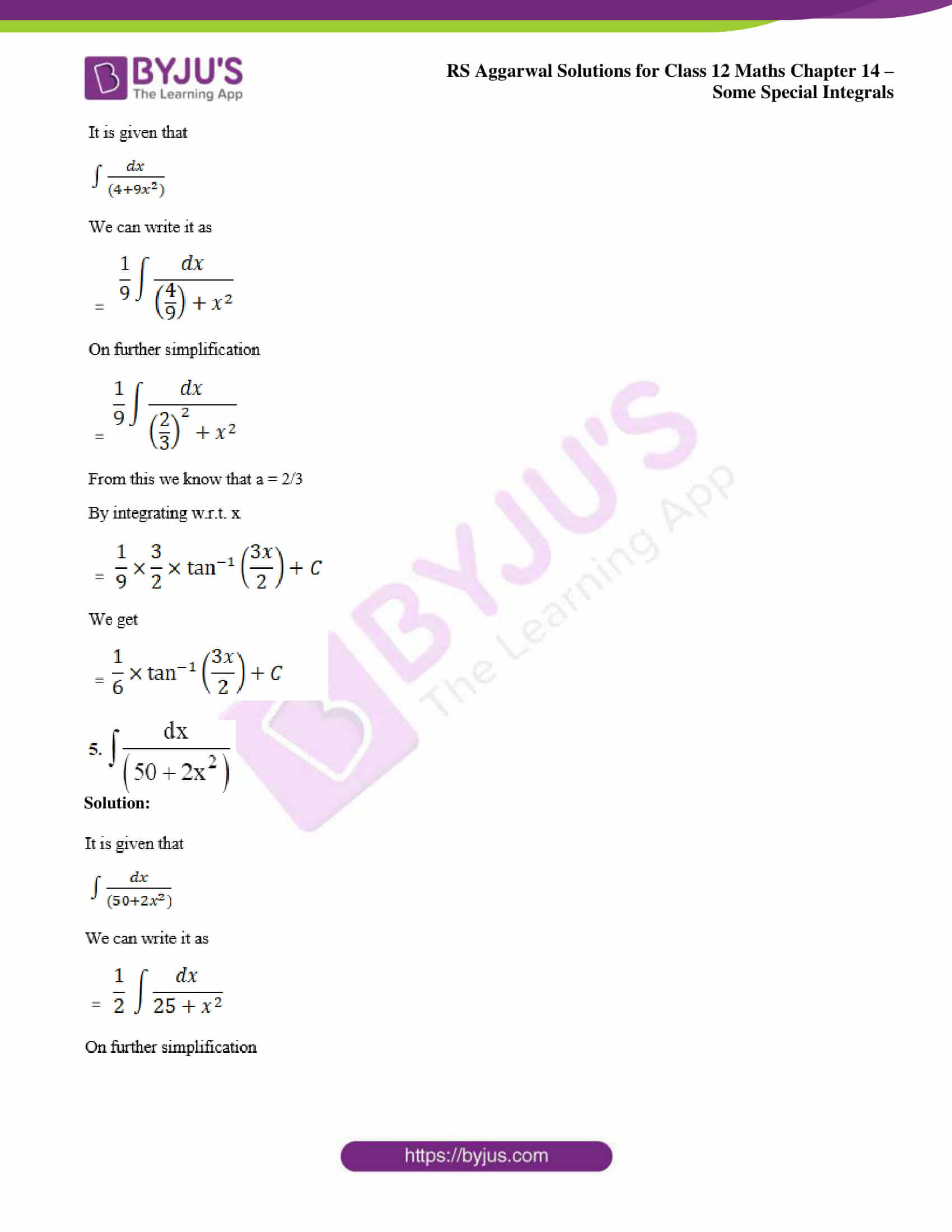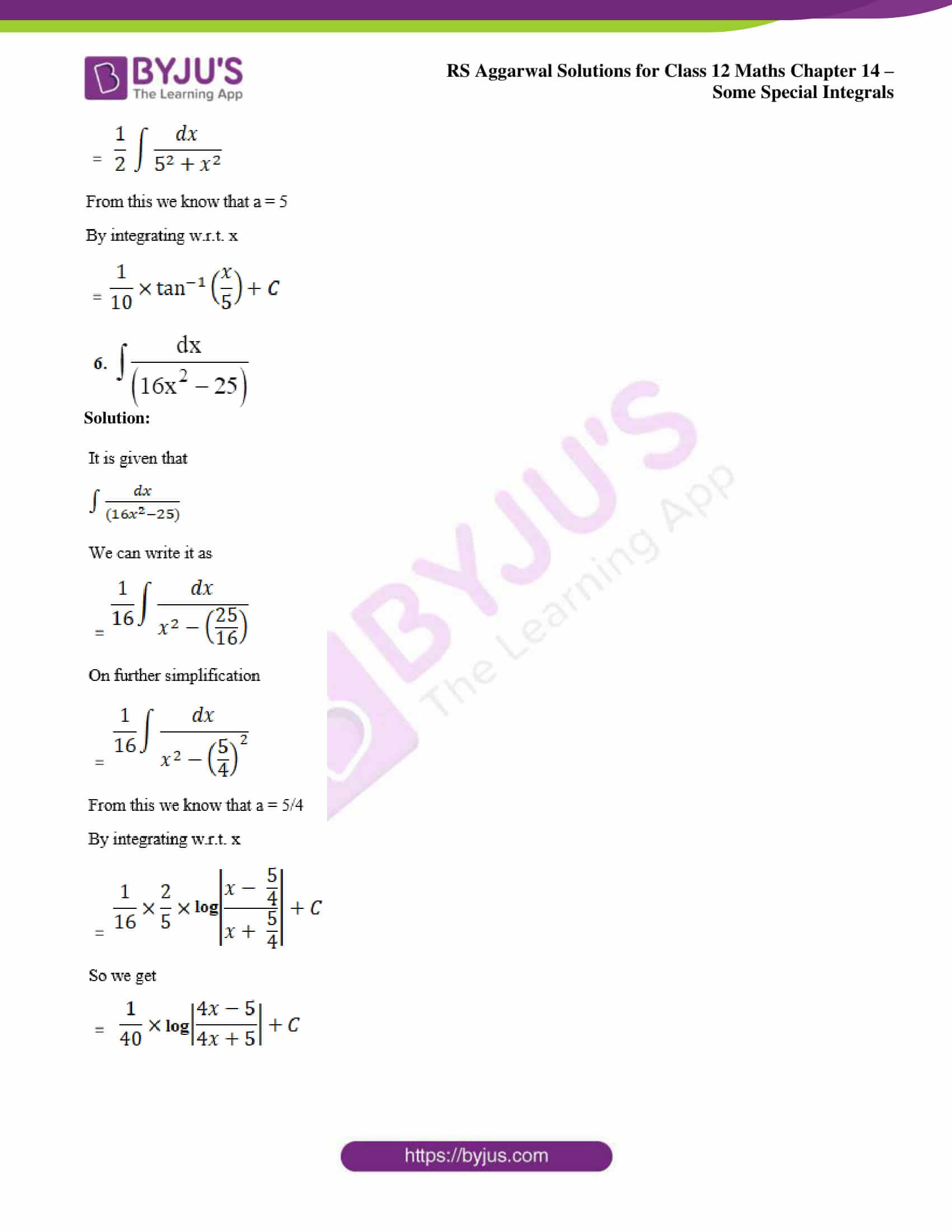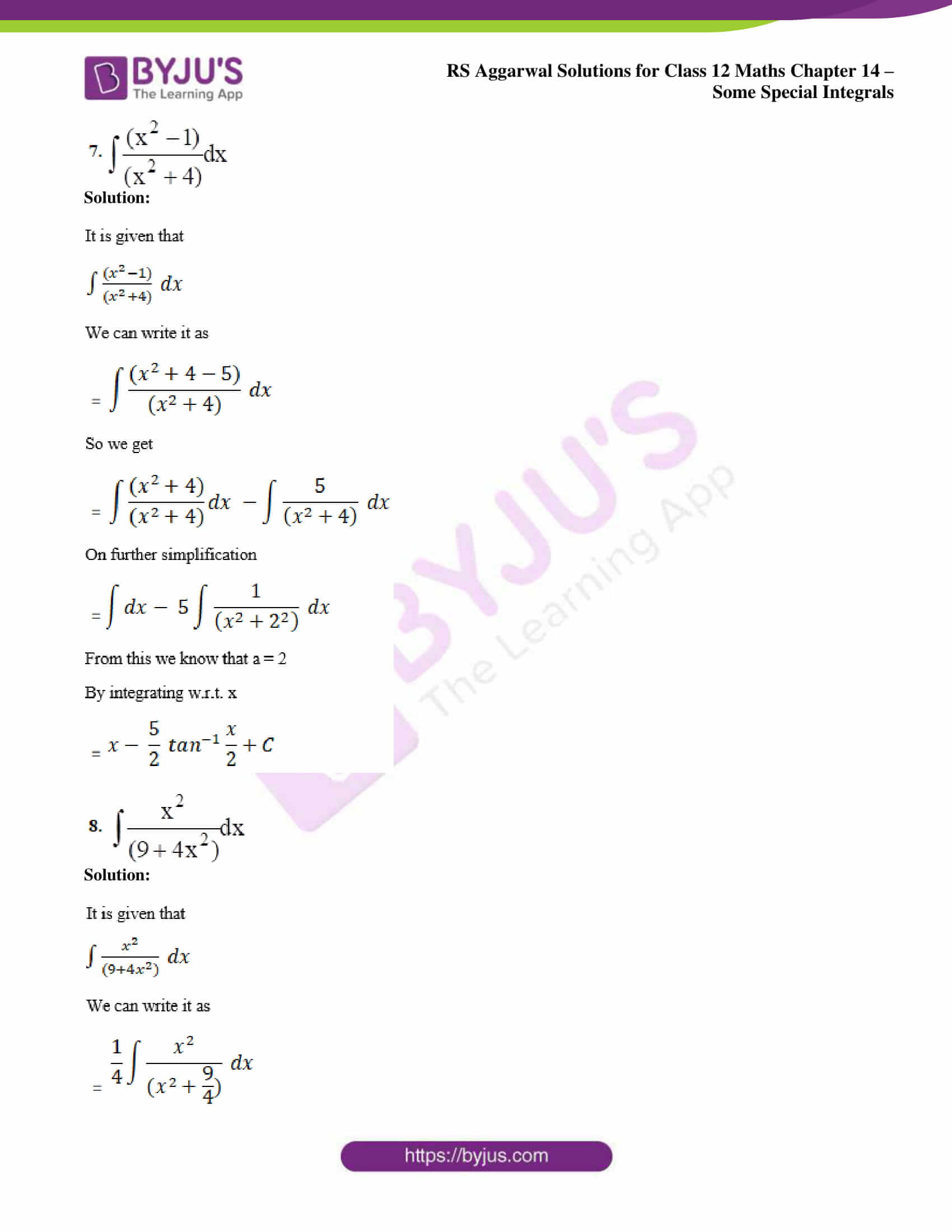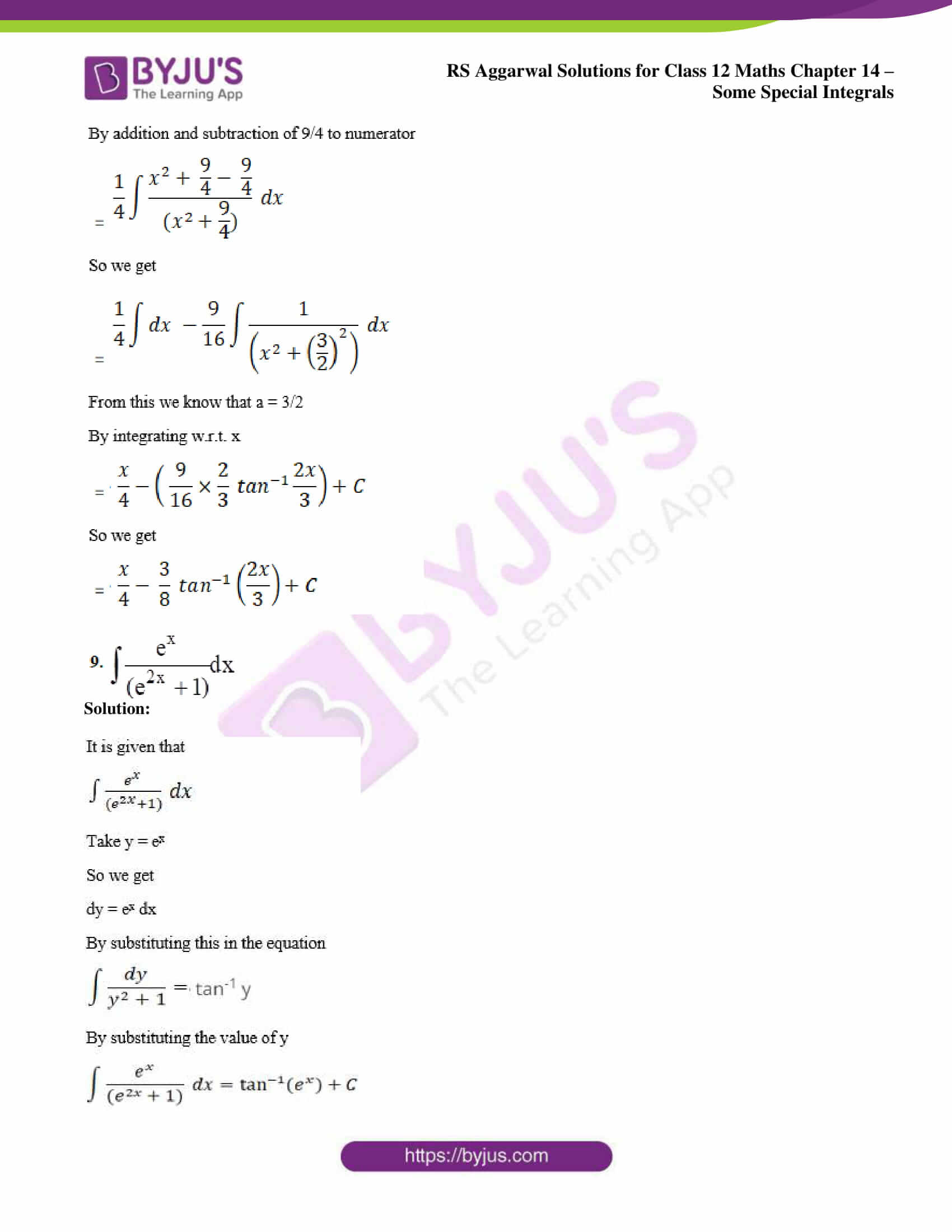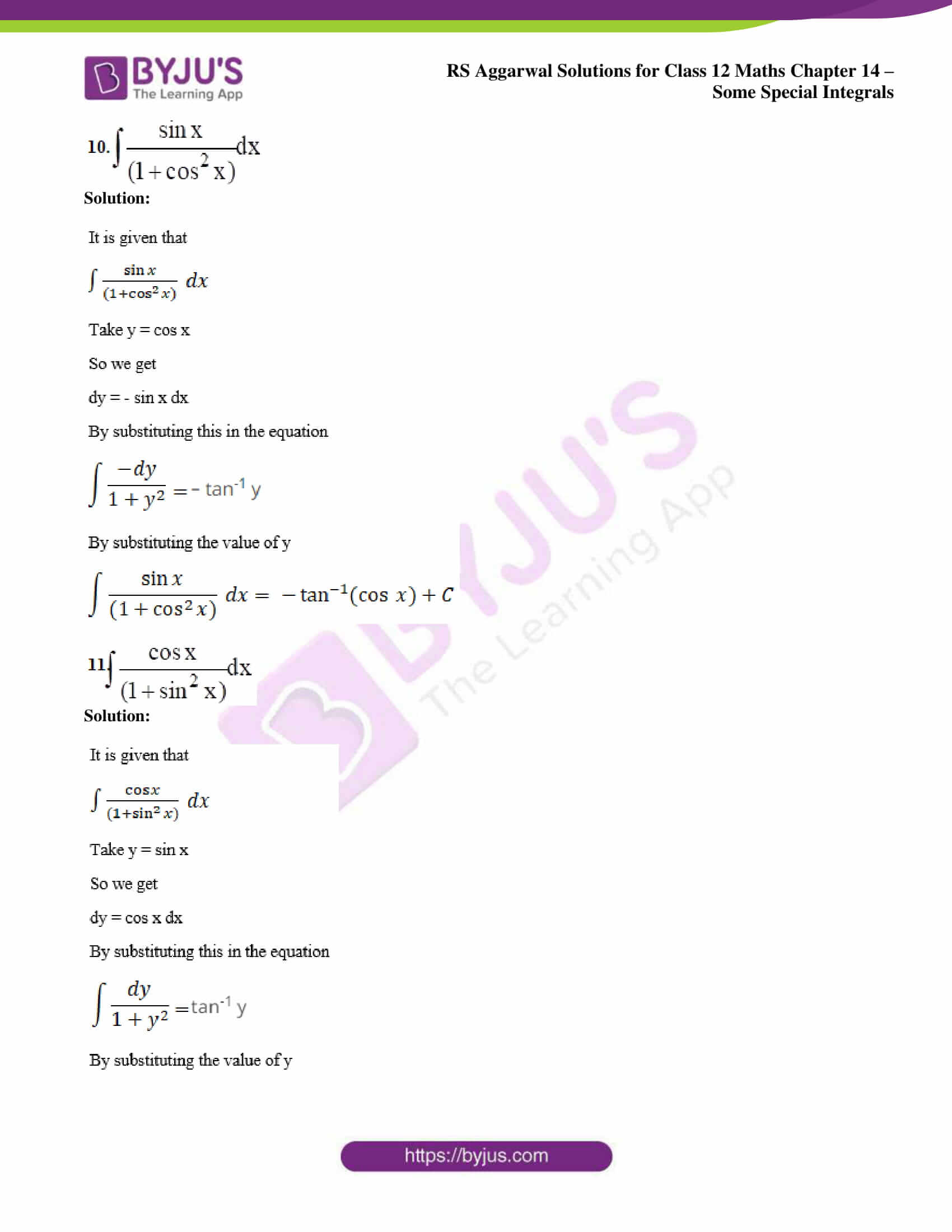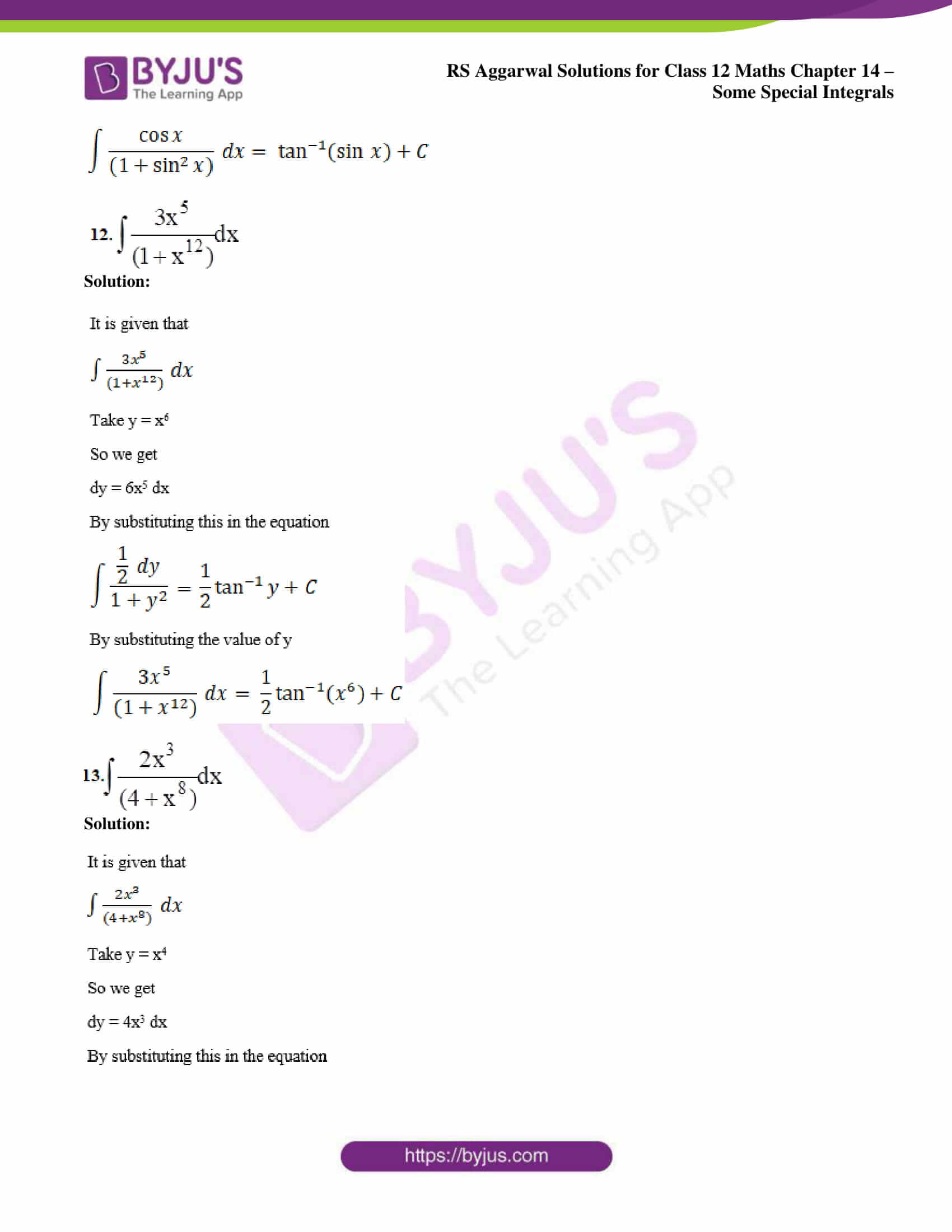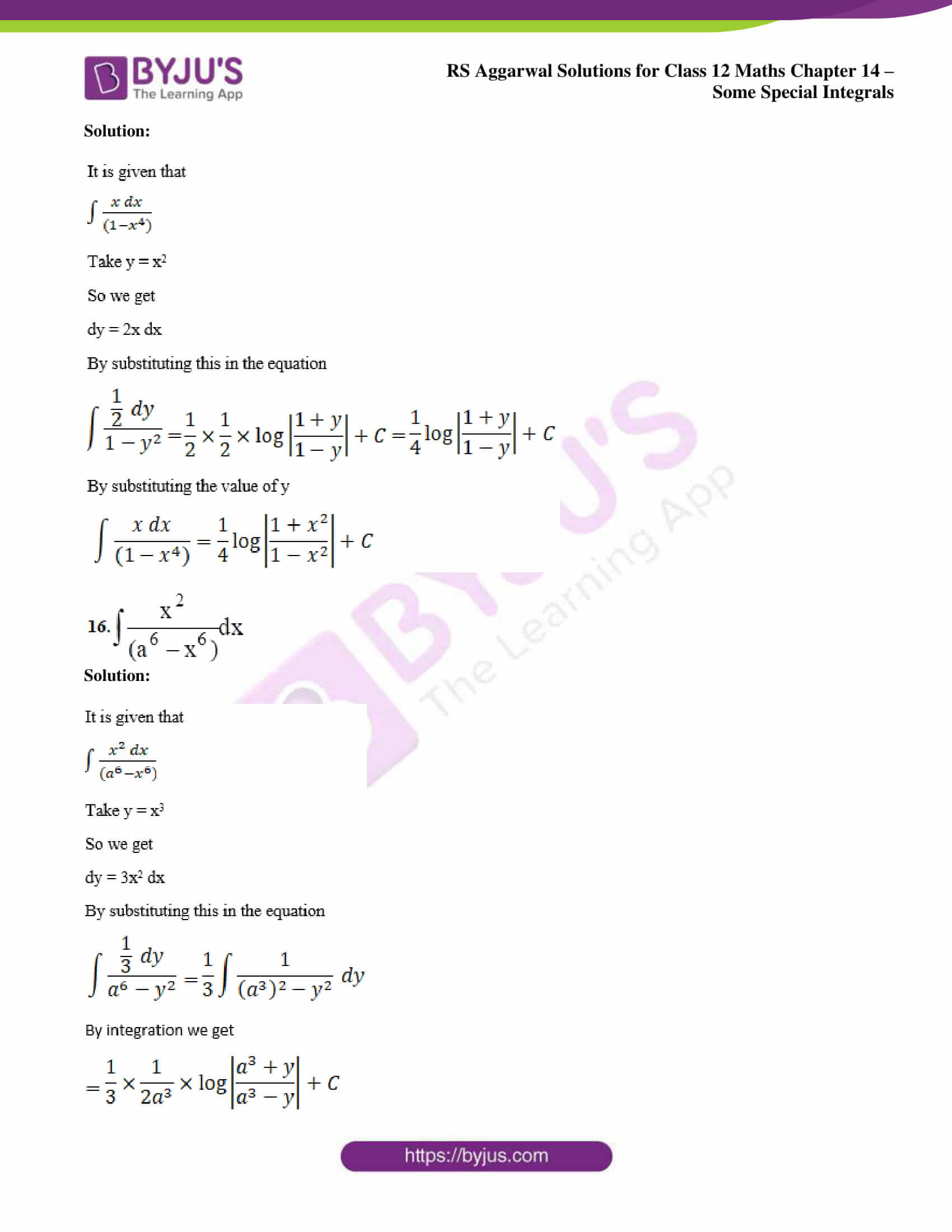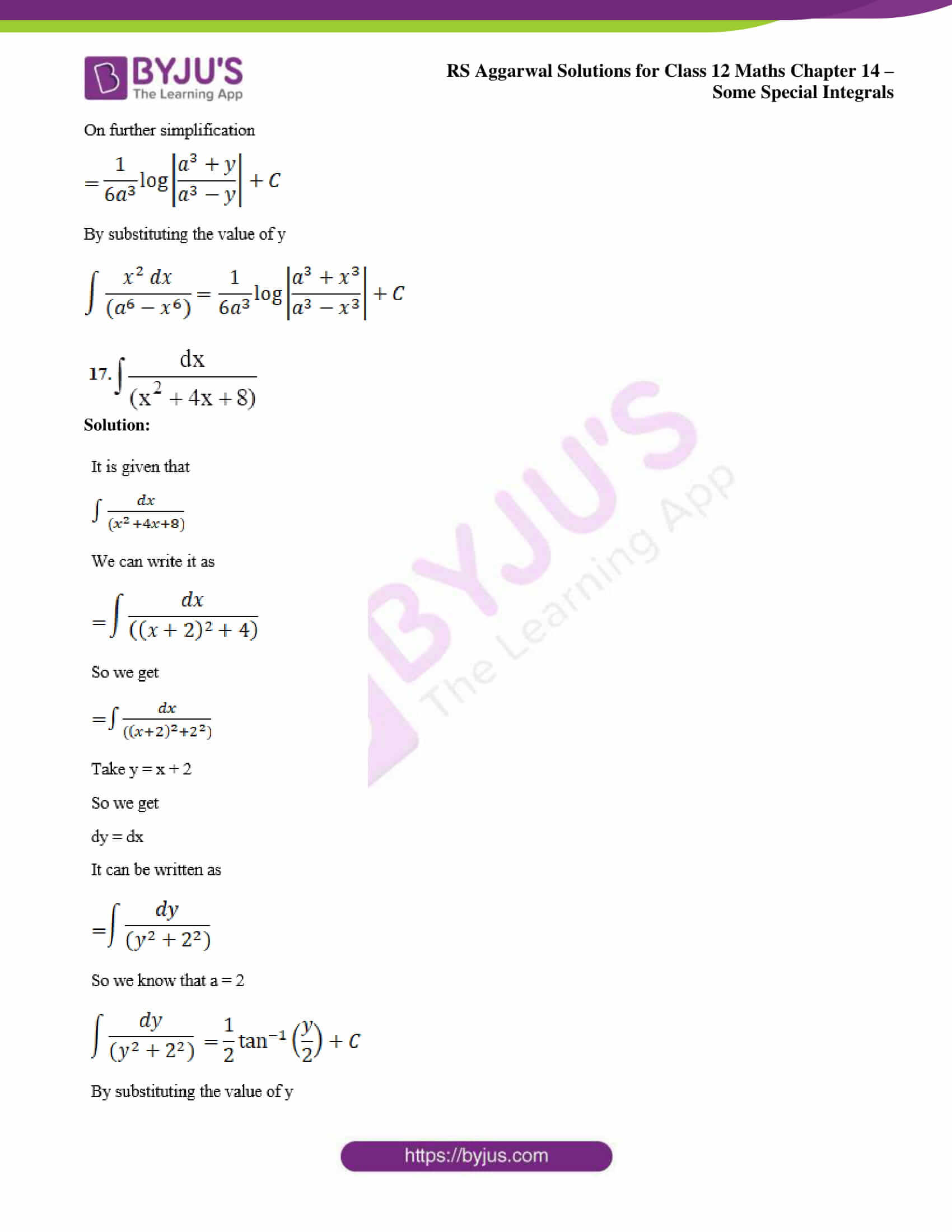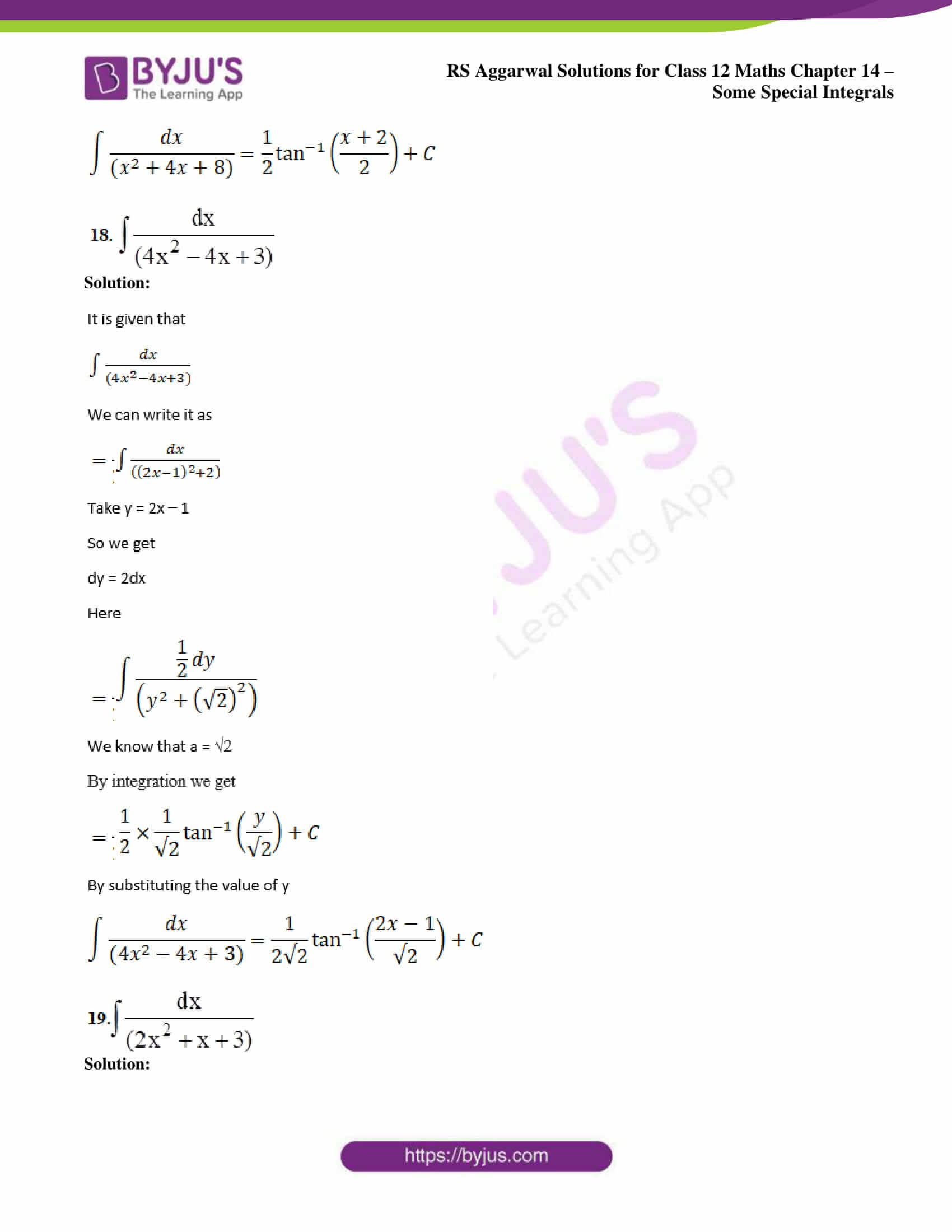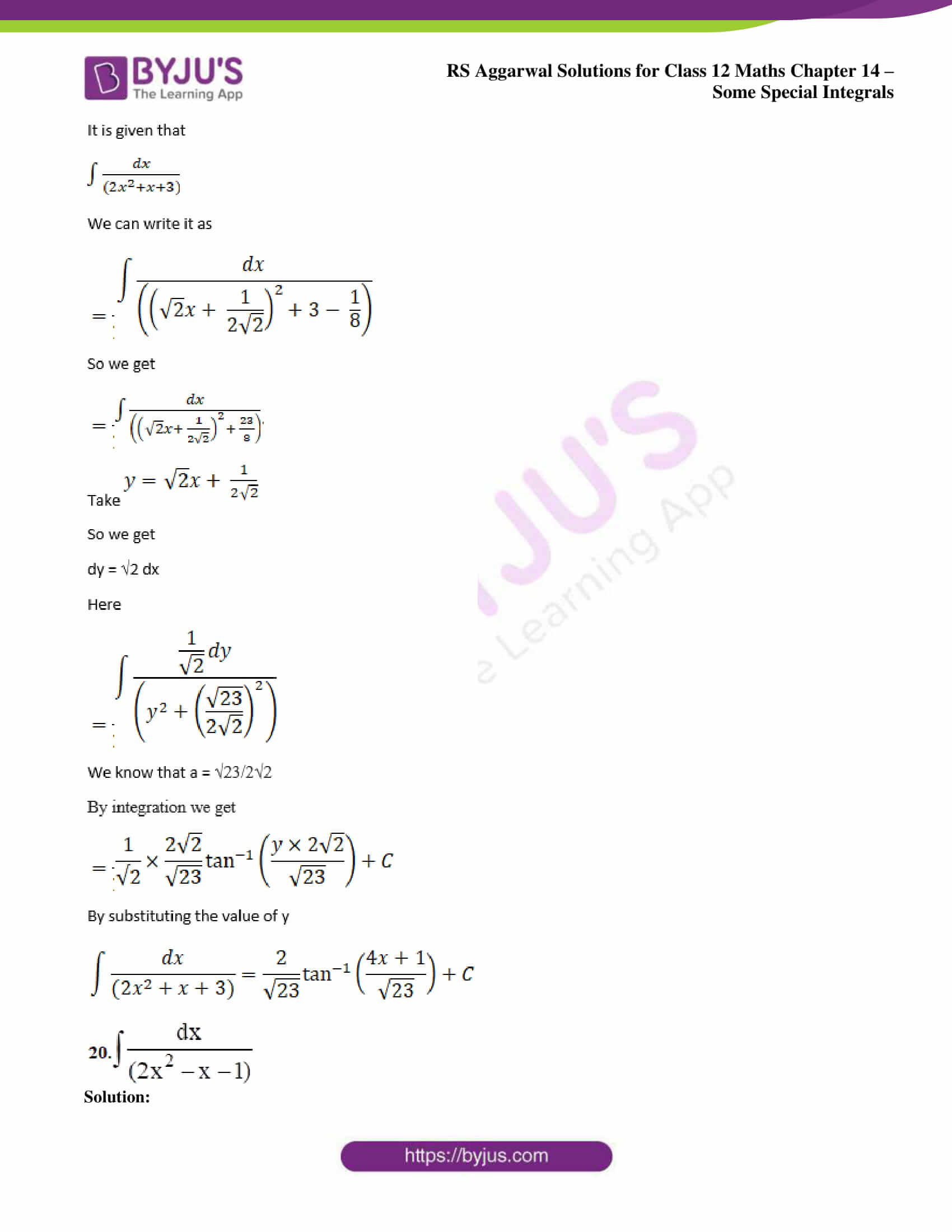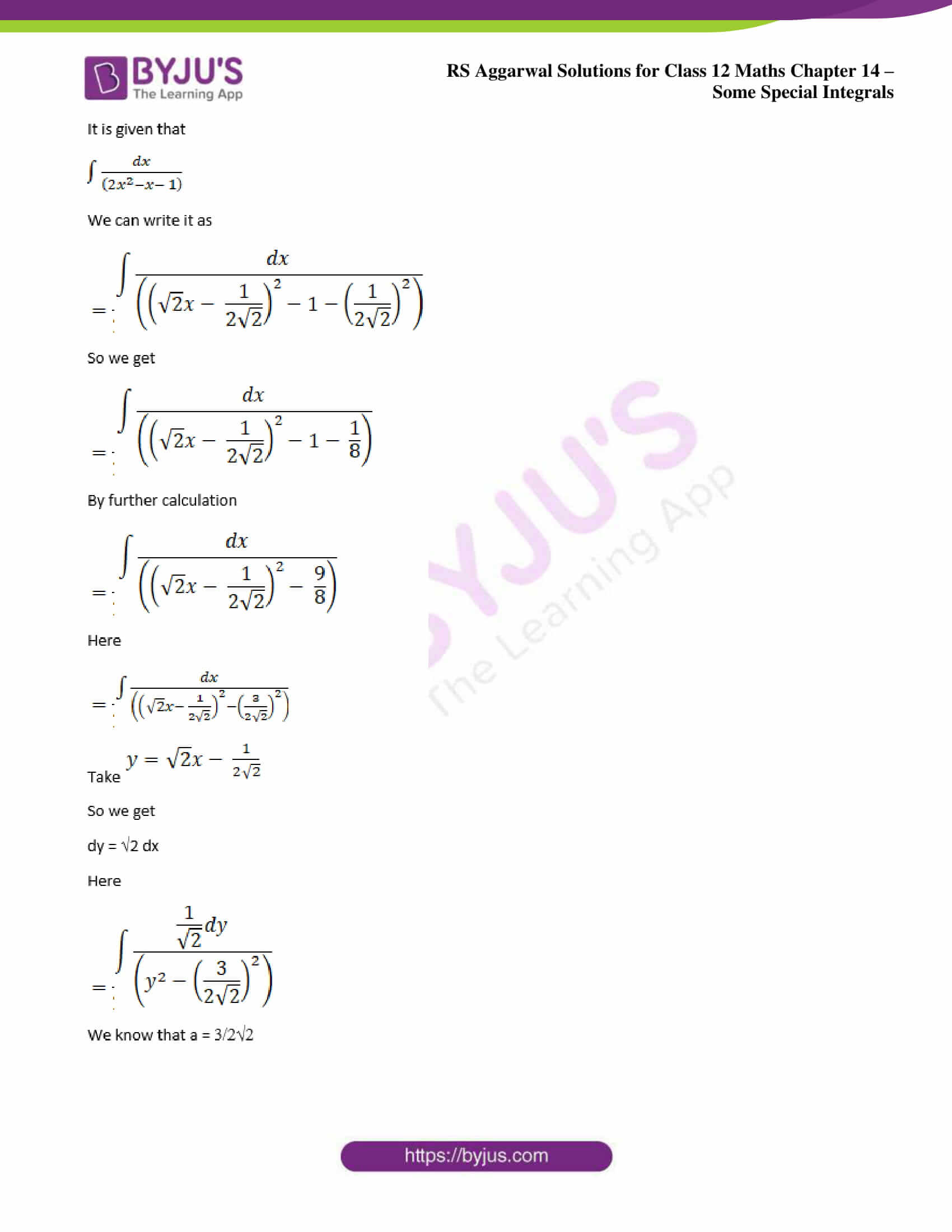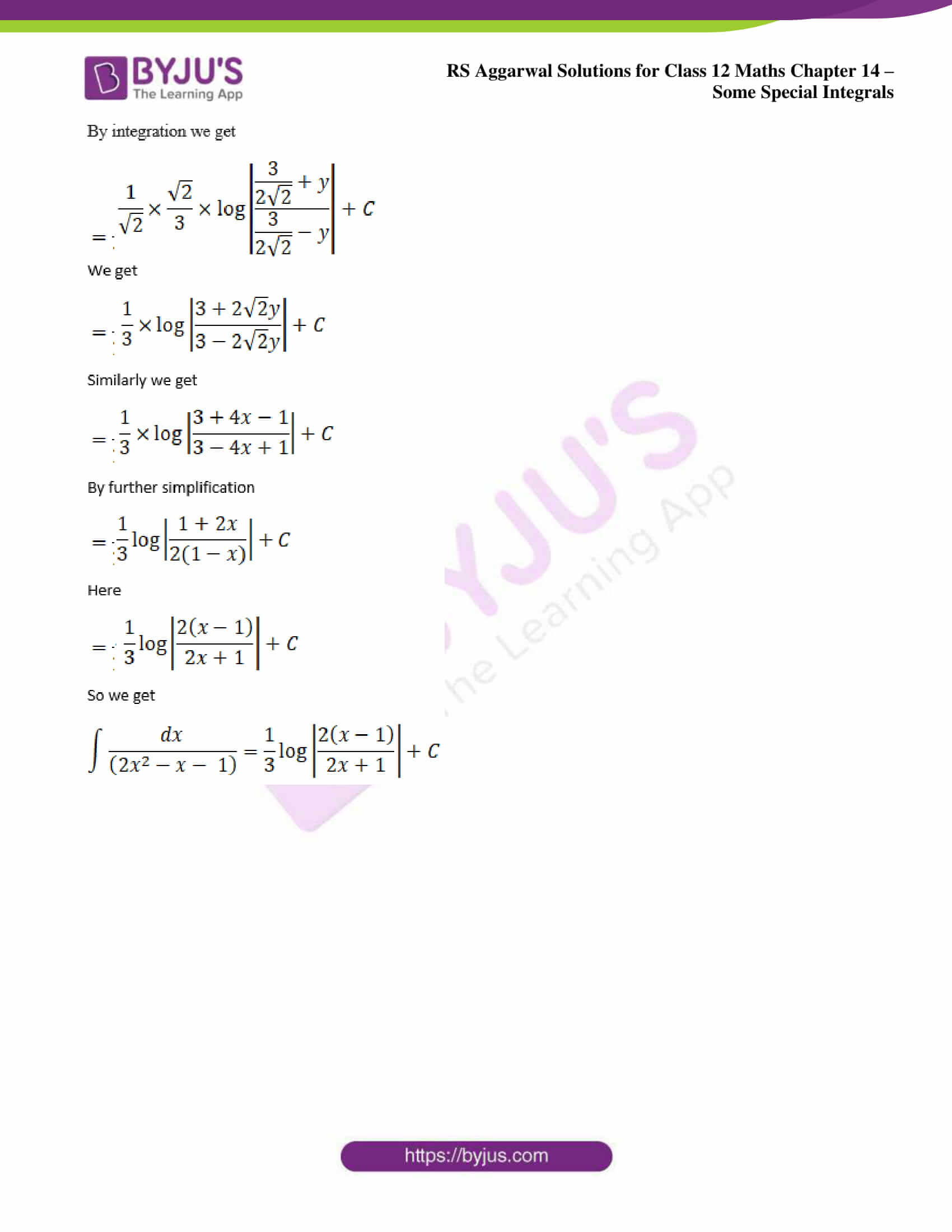### Access other exercise solutions of Class 12 Maths Chapter 14: Some Special Integrals

Exercise 14B Solutions

Exercise 14C Solutions

### Access RS Aggarwal Solutions for Class 12 Maths Chapter 14: Some Special Integrals Exercise 14A

Evaluate: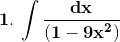Solution: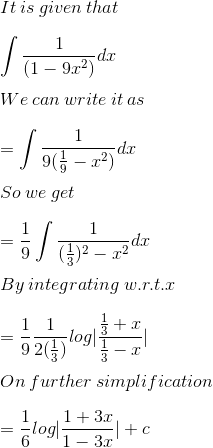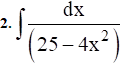Solution: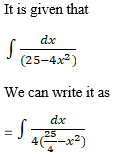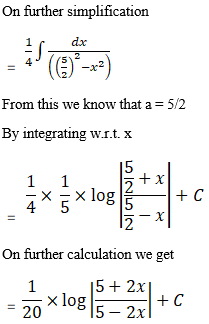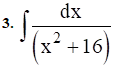Solution: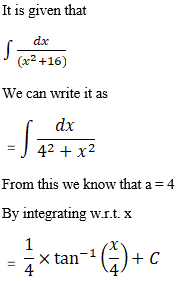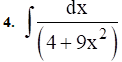Solution: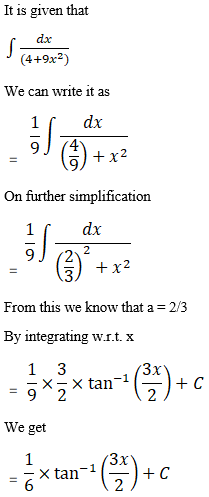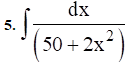Solution: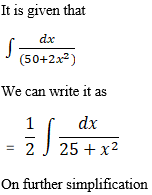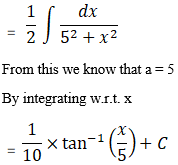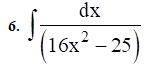Solution: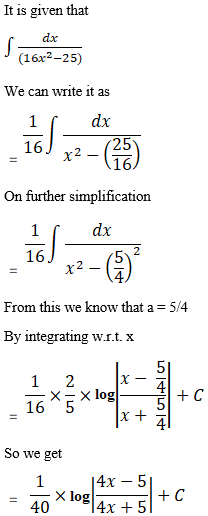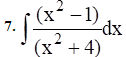Solution: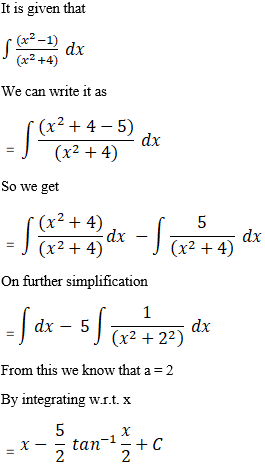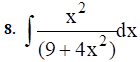Solution: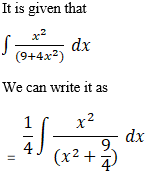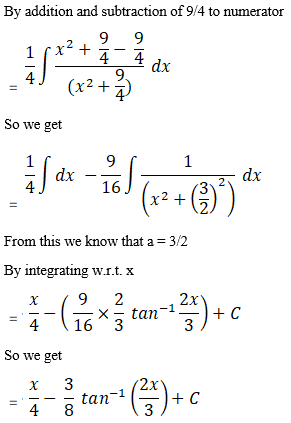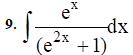Solution: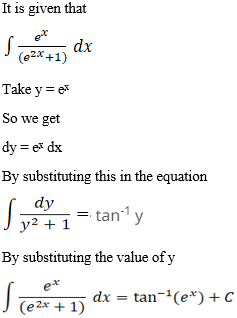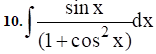Solution: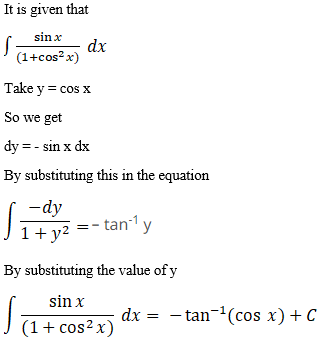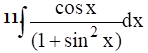Solution: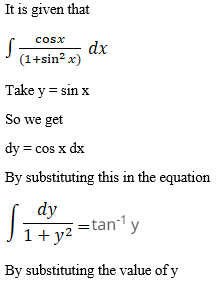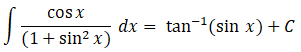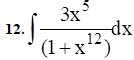Solution: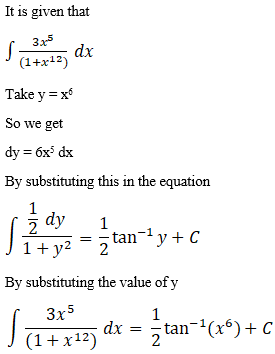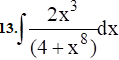Solution: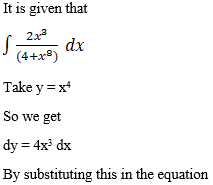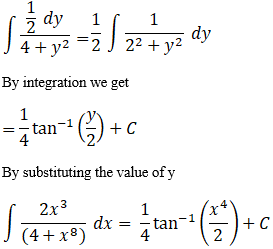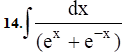Solution: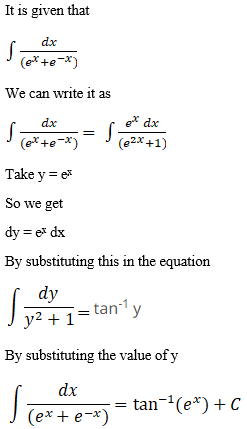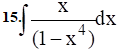Solution: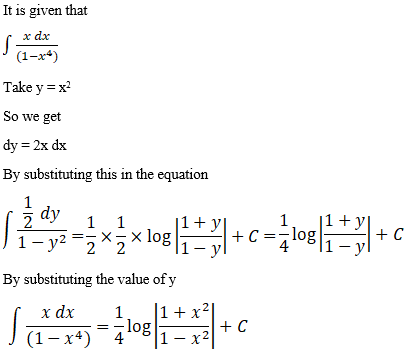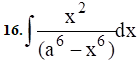Solution: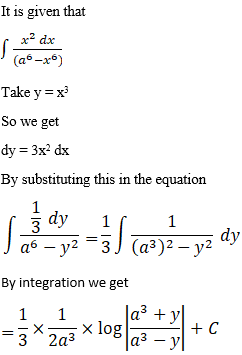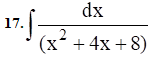Solution: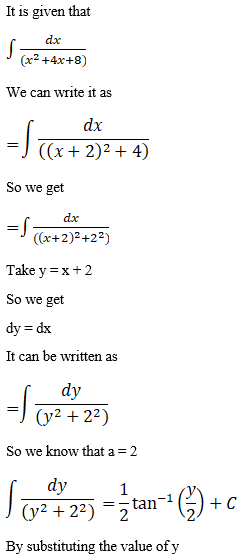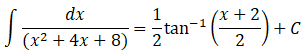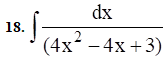Solution: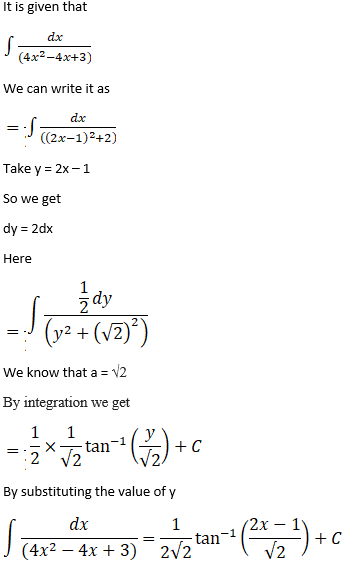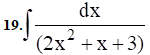Solution: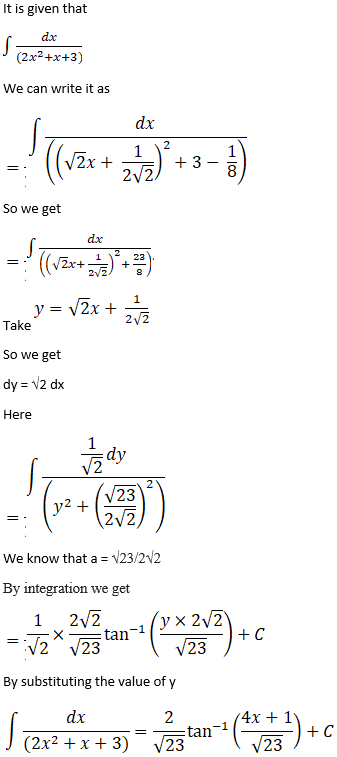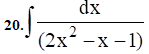Solution: